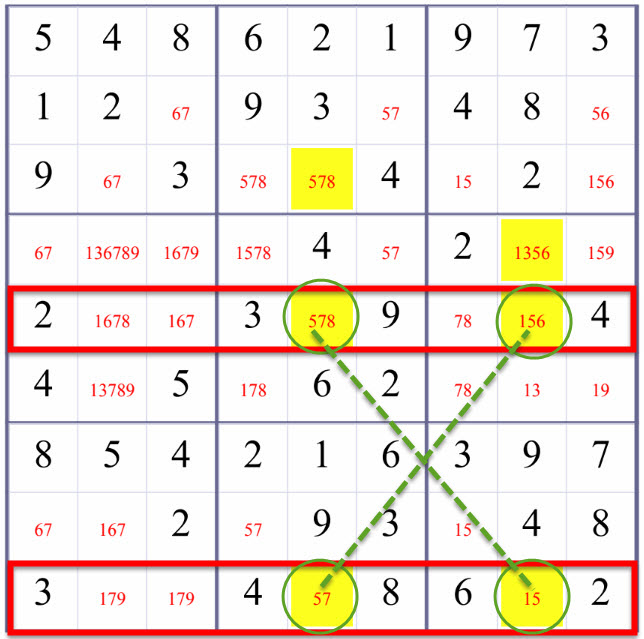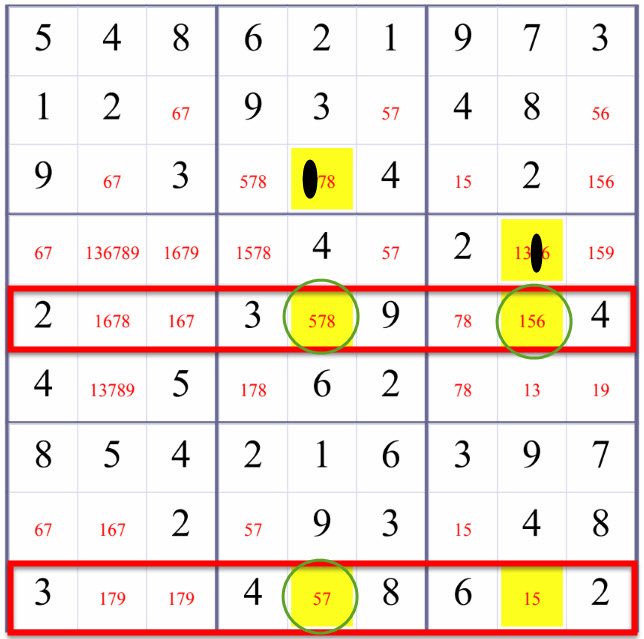Chat

### X-Wing: Advanced Solving Sudoku Technique

X-Wing by createclassicsudoku.com , and www.kristanix.com , and humage.com , and hodoku

X-Wing:

If in two rows all candidates of a certain digit are confined to two same columns, for these two columns this digit must appear within these two rows.

or

If in two columns all candidates of a certain digit are confined to two same rows, for these two rows this digit must appear within these two columns.

Candidate Numbers (or Candidate Values) of an blank/empty square is a list of 'possible values' or candidates for this blank/empty cell.

Take a look at these two possibilities of putting 5 in the fith row and the ninth row, aren't they form a Medium shape of 'X'?These above two possibilites rule out the possiblity that number 5 could be in R3C5 and R4C8 in the fifth column and the eighth column. Therefore, we remove number 5 from R3C5 and R4C8.Above is an example of using DoubleRowsOneDigitCheck(a.k.a. one case of X-Wing) to refine the candidate values of R9C9 (the highlighted squares in the fifth column and the eighth column excluding those at the fith row and the ninth row). Blank/Empty Squares are marked with candidate values in red.

In the fifth row and the ninth row, number 5 are confined to the fifth column and the eighth column. For the fifth row and the ninth row, let us check number 5.

• Either R5C5 and R9C8 take number 5, or
• R5C8 and R9C5 take number 5.

For convenience, in our Sudoku solver, we call

DoubleRowsOneDigitCheck:

If in two rows all candidates of a certain digit are confined to two same columns, for these two columns this digit must appear within these two rows.

DoubleColsOneDigitCheck:

If in two columns all candidates of a certain digit are confined to two same rows, for these two rows this digit must appear within these two columns.

### How to find them?

• Use basic methods to solve the Sudoku until no further blank/empty square can be inferred to be a number using basic methods (Sole Candidate and Hidden Single).
• Write down the candidate values for every blank/empty square.
• Apply interaction methods, Subset, SubsetPosition methods if necessary.
• Check if in two rows all candidates of a certain digit are confined to two same columns, for these two columns this digit must appear within these two rows. If there exist such a digit, remove this digit from candidate values of blank squares in that two columns but not in the two rows.
• Check if in two columns all candidates of a certain digit are confined to two same rows, for these two rows this digit must appear within these two columns. If there exist such a digit, remove this digit from candidate values of blank squares in that two rows but not in the two columns.

##### Solving Sudoku Methods Explained

This article answers questions: What are commonly used solving sudoku methods? What solving Sudoku methods do we use? In what sequences?

##### Basic Solving Sudoku Method: One Choice

a.k.a Sole Candidate, Only Candidate, or Naked Single

##### Basic Solving Sudoku Method: Elimination

a.k.a Hidden Single, Loner

##### Advanced Solving Sudoku Method: Subset2

a.k.a Pair, Naked Pair, or Naked Twins

##### Advanced Solving Sudoku Method: SubsetPosition2

a.k.a Hidden Pair or Hidden Twins

Last updated 3 mins ago

##### Advanced Solving Sudoku Method: Interaction

a.k.a Box/Row Claim or Intersection , or Locked Candidates

##### Solving Sudoku Methods Explained

This article answers questions: What are commonly used solving sudoku methods? What solving Sudoku methods do we use? In what sequences?